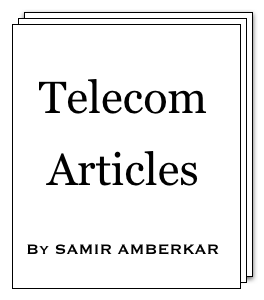online LTE test online C test Updated or New CDF explained5G Page5G Intent (a presentation)5G Intent (article)CV2X PageA Look at CV2X (a presentation)

 About Feedback Information Theory Modulation Multiple Access OSI Model Data Link layer SS7 Word about ATM GSM GPRS UMTS WiMAX LTE 5G Standard Reference Reference books Resources on Web Miscellaneous CV2X Mind Map Perl resources Magic MSC tool Bar graph tool C programming ASCII table Project Management Simple Google box HTML characters Site view counter 7 5 0 , 5 4 0 (May-2010 till Mar-2019)Combined entropy

Combined entropy [Under Information theory > Shannon's paper]

In last article, we mentioned about combined entropy; let us spend little more time in understanding the concept of combined entropy. Say we have two information sources, Ia and Ib, each having its own set of symbols and bit representations or entropies, H(a) and H(b) respectively. What would be their combined entropy i.e. H(a,b) ?

Let us take examples.

Case I: Independent sources

Say Ia has two symbols S1a and S2a having same probability and Ib has three symbols S1b, S2b , S3b and S4b again with same probabilities. A simple example Ia transmission would be S1a S2a S1a S2a S1a ... and that of Ib would be S1b S2b S3b S4b S1b S2b S3b S4b S1b ... If we talk about combining Ia and Ib transmissions, symbols about resultant transmission would be (S1a S1b), (S1a S2b), (S1a S3b), (S1a S4b), (S2a S1b), (S2a S2b), (S2a S3b), (S2a S4b) assuming Ia and Ib are independent. Example of combined transmission (let us call it Ic) would be (S1a S1b) (S1a S2b) (S1a S3b) (S1a S4b) (S2a S1b) (S2a S2b) (S2a S3b) (S2a S4b). Note that all these examples maintain the probabilities that we have assumed and also, probabilities of Ia and Ib symbols have been maintained in example transmission for Ic.

Ia would require 1 bit per symbol for representation, Ib would require 2 bits per symbol, and Ic would require 3 bits per symbol. Now if you look at above example, Ic requiring 3 bits per symbols makes sense as Ic symbols contain both Ia and Ib symbols. As Ia and Ib symbols are produced independently, average bits per symbol required for Ic has to be sum of average bits per symbol required for Ia and that required for Ib.

In other words, H(a,b) = H(a) + H(b).

Case II: Dependent sources

What if Ib is dependent on Ia ? Say Ib symbols S1b& S2b are produced only if symbol produced by Ia is S1a - also Ib produces S3b & S4b only when Ia produce S2a. Probable symbols for combined transmissions would be (S1a S1b), (S1a S2b), (S2a S3b), (S2a S4b). Entropies for Ia and Ib remain same as before, but number of bits per symbol required for Ic would be 2. This is because: for combined transmission, once Ia symbol is produced, we have only two choices from Ib symbols => two choices mean 1 bit per symbol => that makes total as 1 bit per symbol for Ia + 1 bit per symbol for Ib symbol = 2 bits per symbol. What we have used here is called conditional entropy (or equivocation).

In other words, H(a,b) = H(a) + Hb(a).

Above situation can be seen in other direction, say we know symbol produced by Ib, by knowing conditional probability, we can correctly guess the symbol produced by Ia. So we need only transmission of Ib symbols, making combined entropy as 2 bits per symbol. This match with our earlier value.

In the example, we have taken many-to-one dependency (number of Ib symbols dependent on one Ia symbol). There could be many-to-many dependency. Taking care of this, we can write:

H(a,b) = H(b) + Ha(b)

Thus H(a,b) = H(a) + Hb(a) = H(b) + Ha(b) = H(b,a).

Since entropy is about uncertainties, we can recheck above equations from uncertainty point of view.

When information sources are independent, it makes logical sense that uncertainty associated with combined transmissions will be additions of uncertainties associated with individual sources.

When sources are dependent, once symbol for particular source becomes known, uncertainty associated with connected (or dependent) source(s) decreases (lesser number of options to choose from) and vice versa. And so, for dependent sources, conditional probabilities apply !

Above equations seems to be doing just what we expected from thinking of entropy as measure of uncertainty.